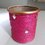# Complex Networks 2

As promised in the first article, I will now try to describe meaning of network or graph. Mathematically, network is simply a set of points (called vertices or nodes) connected by line segments (called edges or links) to each other.network
Figure 1

Historically, concept of graph or network came from great mathematician Leonhard Euler while he was trying to solve the "Seven bridge problem". This is a really interesting story and you must read it.

Now to the mathematics of the networks! We have already introduced the terms 'nodes' and 'links' and I will stick to them. The structure of a network describing a particular complex system can be very different than other networks which describe different physical systems. Even when systems are same, we can't expect exactly same type of connections among the nodes. For example, the detailed structure of connections among neurons for two different persons would be very different. What really matter are the statistical properties of these systems. If the statistical properties of two networks are same, then we will say that they have same 'topology'. (Sometimes, the word 'same topology' is also used to indicate that networks have exactly same structure). Now let me tell you an efficient way to store the topology of a given network (we can't use pictures of networks every time!). Suppose in a given network, there are $n$ nodes and we number them as $1,2,...,n$. Now consider $n\times n$ matrix. In our network, if node $i$ is connected to node $j$, then we will make $(i,j)$th entry of this matrix equal to $1$, otherwise we will make it $0$. This matrix is called 'Adjacency matrix' or 'Connectivity matrix' of the network. The whole information about topology can be stored in adjacency matrix and this makes theoretical and computational analysis of structure of networks way too easy!

In the next article, l will introduce a special network called ER network and related ideas. Please feel free to ask any questions about the ideas which I have presented so far. Also if there is any issue with the way I am presenting, please let me know. Thank you for your response. :)Note by Snehal Shekatkar
7 years ago

This discussion board is a place to discuss our Daily Challenges and the math and science related to those challenges. Explanations are more than just a solution — they should explain the steps and thinking strategies that you used to obtain the solution. Comments should further the discussion of math and science.

When posting on Brilliant:

• Use the emojis to react to an explanation, whether you're congratulating a job well done , or just really confused .
• Ask specific questions about the challenge or the steps in somebody's explanation. Well-posed questions can add a lot to the discussion, but posting "I don't understand!" doesn't help anyone.
• Try to contribute something new to the discussion, whether it is an extension, generalization or other idea related to the challenge.

MarkdownAppears as
*italics* or _italics_ italics
**bold** or __bold__ bold
- bulleted- list
• bulleted
• list
1. numbered2. list
1. numbered
2. list
Note: you must add a full line of space before and after lists for them to show up correctly
paragraph 1paragraph 2

paragraph 1

paragraph 2

[example link](https://brilliant.org)example link
> This is a quote
This is a quote
    # I indented these lines
# 4 spaces, and now they show
# up as a code block.

print "hello world"
# I indented these lines
# 4 spaces, and now they show
# up as a code block.

print "hello world"
MathAppears as
Remember to wrap math in $$ ... $$ or $ ... $ to ensure proper formatting.
2 \times 3 $2 \times 3$
2^{34} $2^{34}$
a_{i-1} $a_{i-1}$
\frac{2}{3} $\frac{2}{3}$
\sqrt{2} $\sqrt{2}$
\sum_{i=1}^3 $\sum_{i=1}^3$
\sin \theta $\sin \theta$
\boxed{123} $\boxed{123}$

Sort by:

Find the third article of this series Here

- 7 years ago

Thanks for #2!

So, for the adjacency matrix $A_{m \times n}$, if the $(m_{i}, n_{j})$ and $(m_{k}, n_{l})$ are two nodes and have link between them, then why do we decide to make the element themselves equal to 1?

For example, if $(m_{i}, n_{j})$ is linked to $(m_{k}, n_{l})$ but not related to $(m_{o}, n_{p})$, then if we consider only $(m_{i}, n_{j})$ and $(m_{o}, n_{p})$, then $(m_{i}, n_{j})$ has a value 0 but if $(m_{i}, n_{j})$ and $(m_{k}, n_{l})$ are considered, $(m_{i}, n_{j})$ has a value of 1.

Have I misunderstood you somewhere?

- 7 years ago

I think you misunderstood this.. we label each node in the network by a single index, we don't specify $(x,y)$ coordinates or something.. that doesn't make sense most of the time. So as per your notation, there will be nodes $m$ and $n$. Now if these two nodes are connected, then $(m,n)th$ entry of $A$ would be $1$, if they are not connected then this entry would be $0$. Hope this answers your question. :)

- 7 years ago

Oh! That clears it. Thanks! Waiting for #3.

That means in my above question if $m^{th}$ and $n^{th}$ node are connected, that makes the $(m,n)^{th}$ element in the adacency matrix 1. If the $m^{th}$ and $p^{th}$ nodes are not connected, that makes the $(m,p)^{th}$ element 0.

- 7 years ago

- 7 years ago

Sir, I hope you are continuing this series! I and many others(I guess) probably want #3 ;)

- 7 years ago

Yes.. soon I will upload 3rd article. Somehow I didn't see the kind of response that I got for my first article. Thank you for your response by the way! :-)

- 7 years ago

Maybe it's possible that all those who responded in #1 are also waiting but are not asking explicitly like I did :-)

But I find #2 more interesting as it involves more technical details (and also 'little' bit maths).

- 7 years ago

Ya Its a gd Article..

- 7 years ago